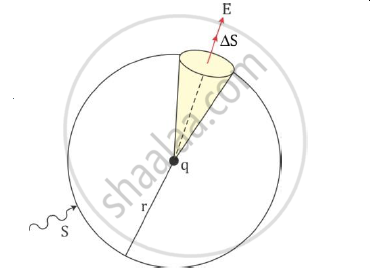# State and Explain Gauss’S Law. - Physics

State and explain Gauss’s law.

#### Solution

Gauss’s law states that the flux of the electric field through any closed surface S is 1/∈ₒ times the total charge enclosed by S

Let the total flux through a sphere of radius r enclose a point charge q at its centre. Divide the sphere into a small area element as shown in the figure.The flux through an area element ΔS is

Deltaphi=E.DeltaS=q/(4piin_0r^2)hatr.DeltaS

Here, we have used Coulomb’s law for the electric field due to a single charge q.

The unit vector hatris along the radius vector from the centre to the area element. Because the normal to a sphere at every point is along the radius vector at that point, the area element ΔS and hatr have the same direction. Therefore

Deltaphi=q/(4piin_0r^2)DeltaS

Because the magnitude of the unit vector is 1, the total flux through the sphere is obtained by adding the flux through all the different area elements.

phi=sum_(all DeltaS)q/(4piin_0r^2)DeltaS

Because each area element of the sphere is at the same distance r from the charge,

phi=q/(4piin_0r^2)sum_(all DeltaS)DeltaS=q/(4piin_0r^2)S

Now, S the total area of the sphere equals 4πr². Thus,

pi=q/(4piin_0r^2)xx4pir^2=q/in_0

Hence, the above equation is a simple illustration of a general result of electrostatics called Gauss’s law

Concept: Gauss’s Law
Is there an error in this question or solution?